﻿ Lipophilicity and QSAR Study of a Series of Makaluvamines by the Method of the Density Functional Theory: B3LYP/6-311++G(d,p)Publications are Open
Access in this journal
Article Versions
Export Article
• Normal Style
• MLA Style
• APA Style
• Chicago Style
Research Article
Open Access Peer-reviewed

### Lipophilicity and QSAR Study of a Series of Makaluvamines by the Method of the Density Functional Theory: B3LYP/6-311++G(d,p)

Sékou DIOMANDÉ, Soleymane KONÉ
Journal of Materials Physics and Chemistry. 2019, 7(1), 20-28. DOI: 10.12691/jmpc-7-1-3
Received October 01, 2019; Revised November 03, 2019; Accepted November 12, 2019

### Abstract

This work has focused on sixteen (16) Makaluvamines listed in the literature to date. It reveals the importance of lipophilicity and other molecular descriptors in the anticancer activity of these molecules. Indeed, these compounds have a high cytotoxicity several cancer cells including: leukaemia, colon, prostate, breast, ovary...... First, we determined the lipophilicity of ten (10) Makaluvamines for which the experimental values of FC50 concentrations on the mentioned cancer cells are known. The lipophilic values are calculated using four methods: Kowwin/LogP, ACD/LogP, A/LogPS and MI/LogP. The experimental values of the FC50 concentrations of these Makaluvamines are known. After that, we did a QSAR study of these ten molecules. This study includes the different lipophilic values calculated with other descriptors estimated at the B3LYP/6-311++G(d,p) theory level. Finally, the theoretical FC50 concentrations of the other six Makaluvamines were predicted with the four models established from the ten molecules.

### 1. Introduction

Over the past quarter century, global marine sources have proven to be a rich source of a wide range of new compounds of medical value 1 such as Makaluvamines. These molecules have shown anti-tumor activities against many cancer cells 2. They have attracted scientists from different disciplines such as organic chemistry, bio-organic chemistry, pharmacology and biology with the aim of developing new drugs. In order to better understand the absorption of drugs into the human body, knowledge of lipophilicity or partition coefficient is essential. Indeed, the water/octanol (LogP) or lipophilic partition coefficient is of crucial importance in predicting pharmacological activity because its transport, its passage through the membranes can be conditioned by its partition between a lipid phase and an aqueous phase 3, 4.

The present study aims to determine the lipophilicity of some Makaluvamines in different forms (neutral, protonated and methylated) on the one hand and to establish a quantitative structure-activity relationship (QSAR) on the other hand. This could help to better optimize the process of intercalation of these molecules in topoisomerase II. The lipophilicity calculations are performed using 4 software programs: KowWIN/LogP, ACD/LogP, A/LogPS and MI/LogP. In addition to lipophilicity, molecular descriptors are used in the research of the QSAR model. These descriptors have been calculated with the DFT method at the B3LYP/6-311++G(d,p) theory level. We will rely on experimental concentration data reducing fluorescence by 50% (FC50) for the research of the QSAR model. This model was developed using XLSTAT software. Each Makaluvamines can be neutral, protonated or methylated, so the models established will be used to predict the FC50 concentrations of structures for which experimental values are not available.

1.1. Theoretical Approach to Lipophilicity

The transport, passage through the membranes and pharmacological activity of a molecule can be conditioned by its division between a lipid phase and an aqueous phase, i.e. its hydrophilic character. This can be quantified by the Octanol-Water partition coefficient, noted (logP), which measures the differential solubility of a solute in these two non-miscible solvents 5. This is an important measure for identifying drug similarity. According to the Lipinski rule, oral drugs must have logP values greater than or equal to -2 and less than or equal to 5 6. It is defined by formula (1):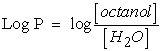(1)

[Octanol] and [H2O] are the concentrations of solute in Octanol and water.

Compounds with log P > 0 values are called lipophilic, and compounds with log P <0 values are called hydrophilic. If the LogP is positive and very high, it expresses that the molecule is more soluble in Octanol than in water. This reflects its lipophilic character, and inversely, if the LogP is negative it means that the molecule is hydrophilic. A null LogP means that the molecule is as soluble in one solvent as in the other.

2.2. Quantitative Relationship Structure Activity (QSAR)

The principle of a QSAR study is to find a mathematical relationship quantitatively linking a biological activity, measured for a series of compounds having the same structural skeleton under the same experimental conditions, with molecular descriptors (physicochemical properties) using statistical methods 3. In this context, a QSAR is designed as a statistical model that links a pool of structural descriptors of a chemical compound with its biological activities.

This can be translated by the following equation: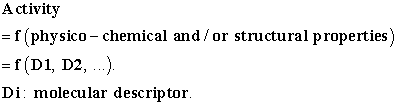The objective of these studies is to analyze the structural data in order to detect the determining factors for the activity studied. The resulting mathematical expression can then be used as a rational way to predict the studied activity for new molecules or molecules for which experimental data are not available. It is then possible for the pharmacochemist to affirm with some confidence that the properties taken into consideration play an important role in the distribution or even the mechanism of action of the active ingredients studied. Similarly, since physico-chemical properties are quantified by QSARs, the pharmacochemist can calculate by anticipation what should be the biological activity of a new analogue and thus focus his synthesis efforts on the analogues assumed to be more active. This reduces the time, cost of synthesis and the number of compounds to be synthesized becomes reasonable 3, 7.

### 2. Materials and Calculation Methods

2.1. Materials

The study focused on the Makaluvamines in Figure 1. Molecules I and A are each considered in neutral and methylated form, we have ten (10) structures, seven (07) of which were used as a training set and three (03) for external validation. Concentrations reducing fluorescence by 50% (FC50) range from 1.2 to 40 µM 8. These FC50 values represent the average of tests on about sixty cancer cells. This range of concentrations allowed us to define a quantitative relationship between cancer activity and theoretical descriptors. By convention, negative logarithms, i. e. logarithms of reciprocal molar concentrations (e. g. log 1/C or pC values), are preferred to obtain higher values for more active compounds (the values in this inverse scale are directly comparable to the pH and pKa values) 9, 10, 11.

• Figure 1. Structures of the studied Makaluvamines (NB: Neutral forms are noted by the refcode X, methylated forms by Xmet)
2.2. Calculation Methods

KowWIN/logP 12, ACD/logP 13 software from ACD/ChemSketch (Freeware) version 2016.2.2, MI/logP 14 and A/logPS 15 were used to calculate the Lipophilicity values. MI/logP and A/logPS are available from vcclab.org. A/logPS is a global method, based on electronic state indices while KowWIN/logP, MI/logP and ACD/logP are substructure methods. KowWIN/logP is an atomic method including steric interactions, H-bonding and polar substructure effects. MI/logP is based on functional groups taking into account intramolecular H-bonds and interactions of charges. The ACD/logP is based on the contributions of individual atoms and structural fragments and on intramolecular interactions between the different fragments. The values are given with an incertitude more or less equal to 0.6 and beyond this value the molecules are considered new for the ACD/logP program database.

2.3. Level of Theory

The relationship between the values of the biological activity of the molecules studied and their molecular structures has been demonstrated by theoretical chemistry calculations with Gaussian 09 16 software. The density functional theory (DFT) 17 was used at the B3LYP/6-311++G(d,p) level for the calculation of molecular descriptors. Indeed, DFT methods are generally known to generate a variety of molecular properties 18, 19, 20, 21, 22 in QSAR studies. These methods increase predictability, reduce calculation time and involve drug conception costs. 23, 24. A molecular geometry optimization calculation is followed by a frequency calculation for each Makaluvamine structure. The global molecular descriptors that we have calculated are as follows: the electronic energy of the molecule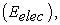the energies of the orbital frontiers (the highest occupied: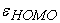and the lowest vacant: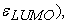first ionization energy (EI), electronic affinity (AE), hardness (η), softness (S), electrophilicity index (ω), chemical potential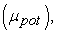electronegativity (χ), dipole moment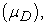polarizability (α), hydrogen-bound acidity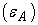and hydrogen-bound basicity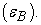The modelling was obtained by the multilinear regression (MLR) method with the softwa re implemented in Excel 25 and XLSTAT 26. The calculation of the partial correlation coefficient between the descriptors studied must be less than 0.70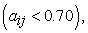this means that these different descriptors are independent from each other 27.

2.4. Estimation of the Predictive Capacity of a QSAR Model

The quality of a model is determined by certain criteria such as the determination coefficient R2, the standard deviation S, the correlation coefficients of the cross-validation Q2CV and the Fischer coefficient F. The statistical indicators R2, S and F concern the adjustment of calculated and experimental values. They describe the predictive ability within the limits of the model and allow the accuracy of the values calculated on the learning set to be estimated 28, 29. The cross-validation coefficient provides information on the predictive power of the model. The R2 coefficient expresses the dispersion of theoretical values around experimental values. The quality of the model is better when the points are close to the adjustment line 30. The adjustment of the points to the line can be evaluated by the determination coefficient.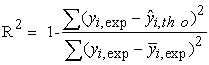(2)

Where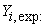Experimental value of anti-cancer activity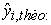Theoretical value of anti-cancer activity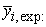The mean value of the experimental values of anti-cancer activity.

The closer the value of R2 to 1, the more the theoretical and experimental values are correlated.

Moreover, the variance σ2 is determined by the relation 1: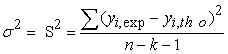(3)

Where k is the number of independent variables (descriptors), n is the number of molecules in the test or learning set and n-k-1 is the degree of freedom. The standard deviation S is another statistical indicator used. It evaluates the reliability and precision of a model: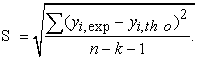(4)

The Fischer coefficient F is also used to measure the level of statistical significance of the model, that is to say the quality of the choice of the descriptors constituting the model.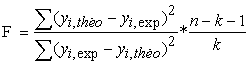(5)

The coefficient of determination of cross-validation Q2CV, makes it possible to evaluate the accuracy of the prediction on the test set and it is calculated by using the following equation: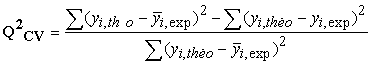(6)

The performance of a model, according to Eriksson and al. 31 is characterized by a value of Q2CV > 0.5 for a satisfactory model and for the excellent model Q2CV is higher than 0.9. A training set of a model will be performing if the acceptance criterion R2-Q2CV < 0.3 is respected. Moreover, the predictive power of a model can be obtained from the value of the ratio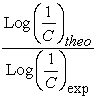for the validation set. The model is acceptable when the value of the ratio of theoretical and experimental activity tends towards unity.

### 3. Results et Discussions

3.1. Lipophilicity

The values of lipophilicity are indicated in the following table:

3.2. Molecular Descriptors

The values of the molecular descriptors are grouped in Table 2.

3.3. QSAR Models

We have developed four (04) QSAR models. Indeed, this is due to the fact that the lipophilicity of each molecule was calculated using four (04) different methods. The results of each method are combined with the other molecular descriptors to search a model. For each model, seven (07) molecules are used for the training set and three (3) for the validation set. The experimental data of FC50 concentrations are relative to the activities of the molecules on various sources of cancer cells of leukaemia, colon, prostate, breast, ovary, renal…. 8.

3.3.1. Model 1

This model was established with lipophilicity obtained from the KOwWIN/LogP software and other molecular descriptors.

3.3.1.1. Model 1 Training and Validation Set

The data used to build this model are presented in Table 3. As for the partial correlation coefficients between the descriptors, they are summarized in Table 4.

Analysis of the data in Table 4 shows that the value of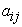between two descriptors is less than 0.70. This shows that the descriptors used in this model are independent.

3.3.1.2. Validation of Model 1

The contribution of a descriptor to anticancer activity in correlation with other descriptors in the regression equation depends not only on the sign of its coefficient but also on its own sign. When the descriptor and its coefficient are the same sign, it improves biological activity. On the other hand, if they have opposite signs, the descriptor reduces the activity. Equation (7) below represents the best model based on the data in Table 3.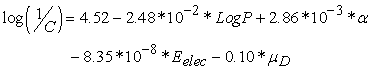(7)

With: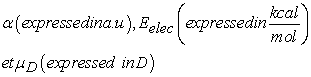R2 = 0.999;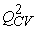= 0.936; F= 385.981; S= 0.789; N= 7;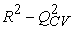= 0.063

Model 1 exalts four (04) descriptors: lipophilicity, polarizability, electronic energy and dipole moment. Figure 2 below shows the coefficients (sign and importance) assigned to these descriptors.

• Figure 2. Coefficients defining the contributions of the four descriptors to anticancer activity in model 1

According to this model, low lipophilicity (LogP<0) contributes to improving the anticancer activity of a molecule in the series. This is the case for the Makaluvamines Amet, Imet, Lmet and Vmet. These molecules are all in charged form. The model predicts that high polarizability (α) will improve the anticancer activity of any molecule in the series. Makaluvamine Lmet has the highest polarizability of the series. Since the electronic energy is always negative, equation (7) shows that the lower the energy of a molecule in the series, the more it improves the activity. The model predicts a decrease in anticancer activity of one tenth of the dipole moment value of any molecule in the series.

The values of the Fischer coefficient (F), the cross-validation coefficient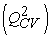et deshow that the model of equation (7) is acceptable.

The external validation of the model was performed with molecules I, E and Lmet. The values of the ratio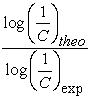of the external validation set are reported in Table 5.

The values of the reportsare close to 1. These results indicate a good correlation between the experimental and theoretical values of the anticancer activity of the molecules studied. Figure 3 shows the regression line between the experimental and theoretical anticancer activity of the training set (blue dots) and the test set (red dots).

• Figure 3. Mean regression of experimental anticancer activity in relation with theoretical anticancer activity for all molecules of the training set and the validation set of model 1

We used values of lipophilicity obtained with the three other methods ACD/LogP, A/LogPS and MI/Log and other molecular descriptors in Table 2 to establish three QSAR models 2, 3 and 4 respectively. Table 6 contains, in addition to the experimental data, the descriptors that are used in the expressions of these models. Table 7 contains the mathematical expressions of the said models and the values of the different coefficients: determination R2, standard deviation S, correlation of cross-validation Q2CV and Fischer F.

For these three models as for model 1,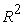is close to 1. The experimental and theoretical values correlate well. All values of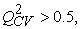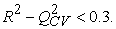The training set of each model is efficient. The high values of the F coefficient also reflect a good statistical significance of the model and the quality of the choice of the descriptors that make up the model.

The partial correlation coefficients between the descriptors of models 2, 3 and 4 are grouped in Table 8.

Analysis of the data in this table shows thecoefficient between two descriptors of a given model is less than 0.70. This shows that these descriptors in the model are independent.

3.3.1.3. Validation of Models 2, 3 and 4

Model 2 presents the contribution of three (03) descriptors to the anticancer activity of the series of compounds. These are lipophilicity, polarizability and electronic energy. As for model 3, two descriptors, lipophilicity and polarizability, contribute to the anticancer activity. Model 4 correlates four (04) descriptors to predict the anticancer activity of the molecules studied. These are lipophilicity, first ionization energy, hardness, and dipole moment.

Figure 4 shows the coefficients assigned to the different descriptors of models 2 (4a), 3 (4b) and 4 (4c).

Models 2 and 3 show that negative lipophilicity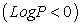improves the activity of a Makaluvamine. However, if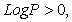activity decreases. A high value of polarizability contributes to increasing the activity of the molecules in the series studied. According to model 2, the high values of electron energy, which are lower in absolute values, should allow a better anticancer activity of these molecules. Model 4 shows that a high value of lipophilicity and also the first ionization energy strongly contributes to improve the anticancer activity of a molecule in the series studied. For a good contribution to this activity in such a molecule, the hardness and dipole moment must be low. The molecule must be slightly polar and soft. External validation was performed with molecules I, E and Vmet and for models 2 and 3 then with molecules I, E and Lmet for model 4. The values of the ratioof the external validation set are shown in Table 9.

• Figure 4. Coefficients attributed to the different descriptors of models 2, 3 and 4
• Figure 5. Mean regression of experimental anticancer activity in relation with theoretical anticancer activity for all molecules in the training set and the validation set of three models 2, 3 and 4

These plots show a good correlation between the experimental data and the results of theoretical calculations of the anticancer activity of the molecules studied.

All four established models show the contribution of lipophilicity to anticancer activity, three of them show the contribution of polarizability. The dipole moment and electronic energy appear in two of the models. By referring to the quality parameters, models 2 and 3 are the best. They confirm the importance of two descriptors in predicting and improving anticancer activities: lipophilicity and polarizability. It should also be noted that according to the Lipinski rule, lipophilicity (LogP) is important for the identification of similarity with a drug 27. Polarizability is also one of the parameters that reflects the molecular properties related to hydrophobicity and therefore to biological activities 5.

3.3.2. Prediction of Anticancer Activity: Theoretical Determination of FC50 concentrations

For all forms (neutral, protonated and methylated) of each Makaluvamine C, H, K(P) and O in Figure 6 and those of the compounds in Figure 1 for which experimental FC50 concentration values are not available, we made a theoretical estimate. The four models established were applied.

The four values of lipophilicity (KowWIN/logP, ACD/LogP, A/LogP and MI/LogP) of the different forms of these Makaluvamines and the different molecular descriptors that are exalted in the models have been calculated. The application of the models led to the results in Table 10.

These data cannot be compared due to the lack of experimental values. These are now new data to be exploited. Despite the differences between the neutral, protonated and methylated forms, it is models 2 and 3 that give the best agreement with the experimental values of FC50 of Makaluvamines in the training and validation set of the established models. The experimental FC50 concentrations of Makaluvamines Lmet and E show that these molecules are the most effective. It is important to note that the theoretical values, derived from our calculations, which are generally low for forms L, LH+, EH+ and Emet always reflect their efficacy.

• Figure 6. Structures of Makaluvamines without experimental FC50 values

### 4. Conclusion

The QSAR study carried out on a series of Makaluvamines made it possible to identify major descriptors in the prediction of the anticancer activity of the family of the said molecules. These include lipophilicity (LogP) and polarizability (α). In addition to these two descriptors, the dipole moment can also be decisive. When it appears as a descriptor in a model, the molecule must be low polar. The prediction of the theoretical anticancer activity of molecules without experimental FC50 has demonstrated the efficacy of L, LH+, EH+ and Emet molecules in Models 2 and 3. Thus, this study opens new ways in the conception of new molecules with improved biological activities in the effective fight against certain cancers

### ReferencesThis work is licensed under a Creative Commons Attribution 4.0 International License. To view a copy of this license, visit http://creativecommons.org/licenses/by/4.0/